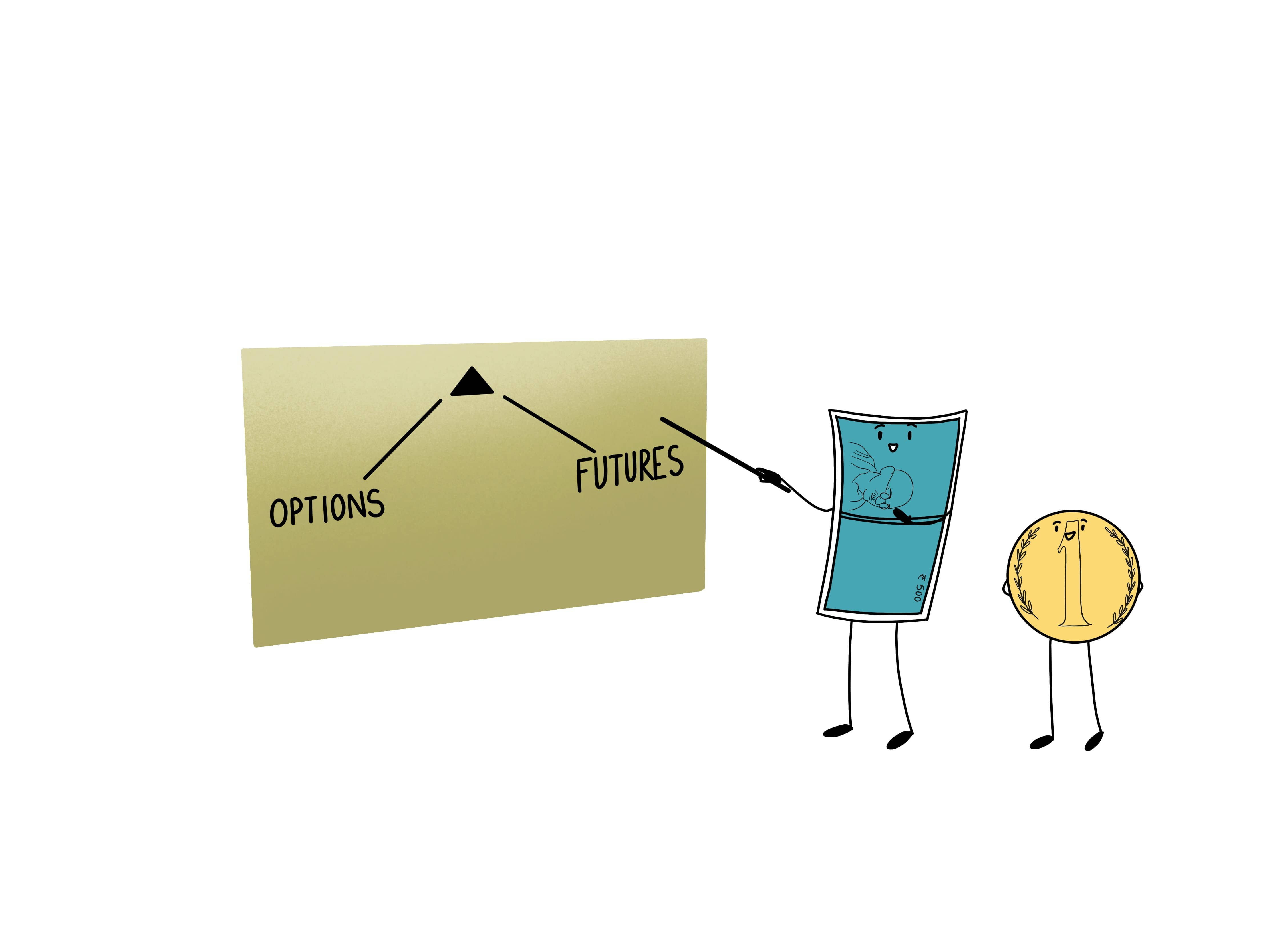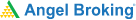# Delta: Relationship between Delta, Options and FuturesRemember our friends Option Greeks? Option Greeks measure how the option value changes in various variables like market price, interest rates, volatility and time to expiry. Let’s dive deeper into Option Delta.

## Role of Option Delta

Option Delta is used to measure the degree to which the price of an option responds to the changes in market price of the underlying. For instance, if one is looking at a Reliance 1,500 call option expiring on the last Thursday of April 2020 then Delta will be used to measure how the option price of this particular contract changes with respect to that of the price of Reliance spot. An option delta of a call option will vary from 0 to 1 while the option delta of a put option will vary from 0 to -1. On the other hand, for an out-of-the-money option, it will be closer to 0. Effectively, call options will have a positive delta while put options will have a negative delta.

### How can traders make sense of Delta for trading options?

Different scales are used to measure Option Delta. Some traders use a scale of 0 to 1, while others use a scale of 0-100. This means that a Delta value of 0.07 will mean 70 on the other scale, however, the underlying concept will remain the same.

A Delta value of 0.05 shows the sensitivity of the price of the option to shifts in the price of the underlying. If the Reliance stock moves up by Rs. 20 then the price of the call option with a delta of 0.7 will move up by Rs 12. Similarly, the price of a put option with Delta of -0.70 will move lower by Rs 12 since put options are negatively related to the stock price.

### What traders must remember about the Option Delta

1. Delta can also be seen as the probability of the option expiring in the money (ITM). This is why an ATM option has a delta of around 0.5, deep ITM options have delta closer to 1 and deep OTM options have delta closer to 0.
2. Delta is positive for call options and negative for put options. A rise in price of the stock is positive for call options but negative for put options. A positive delta means that you are long on the market and a negative delta means that you are short on the market.
3. Delta changes over a period of time. It depends on factors like volatility, interest rates and time to maturity.

Learning & Earning is now super simple₹ 0 Equity Delivery

No Hidden Charges₹ 20 Per Order For Intraday

FAQ,Currencies & CommoditiesZERO Brokerage*

on ALL SegmentsFREE Margin

Trade Funding

### Relationship between Delta, Options and Futures:

Let’s assume that we have a call option priced at 1.00 and it has a .50 delta. This indicates that whatever the change of the underlying future is, the option will move by 50% of that change. The underlying futures product moved from 96 to 97.5, which is a 1.5 point move. Therefore, our option’s premium will now change by 50% of 1.5 ie .75.  This makes the option’s new price 1.75.

#### Wrapping Up

Here are a few points to remember about delta:

1. Calls always have positive delta between 0 and 1.00, while puts always have negative delta between 0 and -1.00.
2. The delta of a futures contract is 1.00.
3. Traders commonly refer to the delta without the decimal point. Therefore, a .40 delta is commonly referred to as a 40 delta.
4. Being long a call will result in positive Delta, while being short a call results in negative Delta.
5. On the other hand, being long a put results in negative Delta, while being short a put results in positive Delta.
6. The absolute value of the Delta also tells the approximate probability that the option will finish in-the-money.
• For instance, if the option has a delta of 20, it suggests that it has a 20% chance of finishing in-the-money.  A delta of 50 suggests it has a 50-50 chance of finishing in-the-money.
7. If an options delta is less than 50 it is said to be out of the-money.  If the delta is greater than 50 the option is said to be in-the-money.  If the delta is equal or close to 50 the option is said to be at-the-money.
8. The delta is used in calculating hedge ratios to establish a neutral or delta hedged position using the underlying futures.
9. Delta is dynamic and changes with movement in the underlying. This means delta neutral ratios and other hedge ratios using options are also dynamic and change too.

#### A Quick Recap

1. Option Greeks measure how the option value changes in various variables like market price, interest rates, volatility and time to expiry.
2. Option Delta is used to measure the degree to which the price of an option responds to the changes in market price of the underlying.
3. Different scales are used to measure Option Delta. Some traders use a scale of 0 to 1, while others use a scale of 0-100.
4. Delta can also be seen as the probability of the option expiring in the money (ITM).
5. Delta is positive for call options and negative for put options.
6. Delta changes over a period of time. It depends on factors like volatility, interest rates and time to maturity.Test Your Knowledge

Take the quiz for this chapter & mark it complete.

Comments (0)

Add Comment

Get Information Mindfulness!

Catch-up With Market

News in 60 Seconds.The perfect starter to begin and stay tuned with your learning journey anytime and anywhere.Ready To Trade? Start withOpen an account